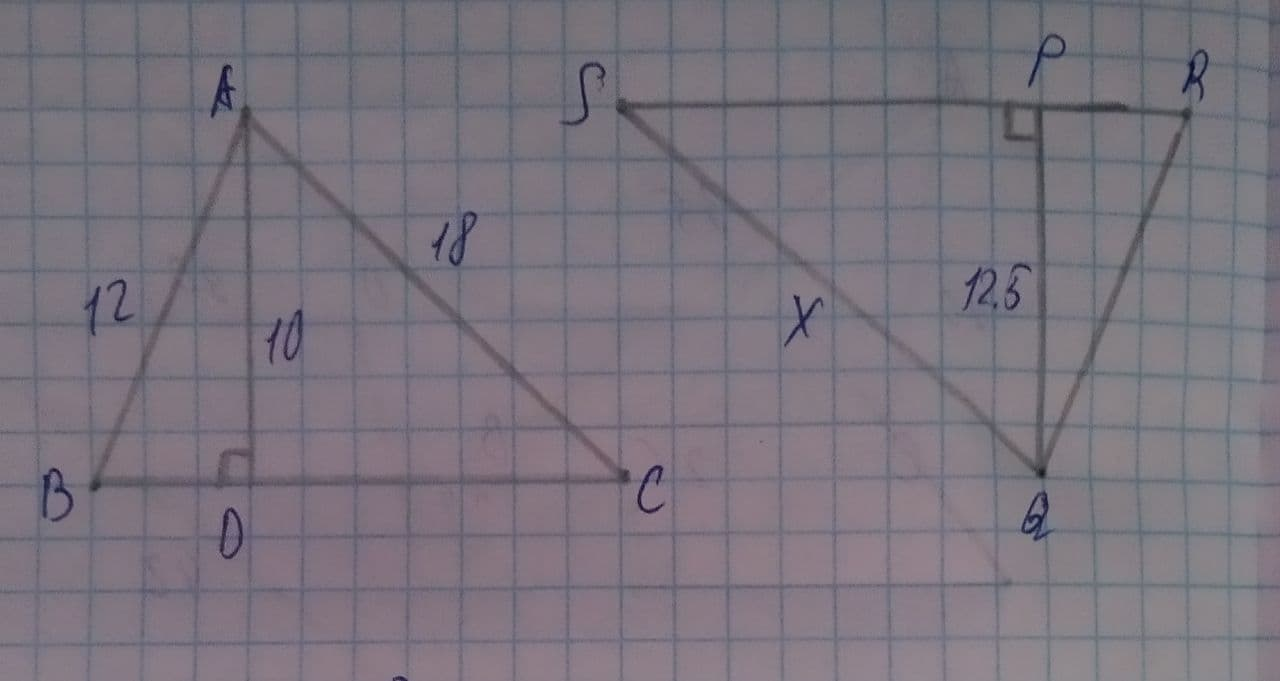# \triangle ABC\sim \triangle QRS what is the value of x?nicekikah 2021-08-03 Answered
Given $\mathrm{△}ABC\sim \mathrm{△}QRS$ what is the value of x?You can still ask an expert for help

• Questions are typically answered in as fast as 30 minutes

Solve your problem for the price of one coffee

• Math expert for every subject
• Pay only if we can solve ithosentak
Given that $\mathrm{△}ABC\sim \mathrm{△}QRS$
by the corresponding part of similar triangles

now in
here $\mathrm{\angle }ADC=\mathrm{\angle }QPS$ (each ${90}^{\circ }$)
$\mathrm{\angle }C=\mathrm{\angle }S$ (from above)
using angle angle similarity criteria of triangle
$\mathrm{△}ADC\sim \mathrm{△}QPS$ (by angle angle similarity)
by the corresponding part of similar triangles
$\frac{SQ}{CA}=\frac{AD}{QP}$
$⇒\frac{x}{18}=\frac{10}{12.5}$
$⇒x=\frac{10×18}{12.5}=14.4$
$⇒x=14.4$